# How to print an entire Pandas DataFrame in Python?

Data visualization is the technique used to deliver insights in data using visual cues such as graphs, charts, maps, and many others. This is useful as it helps in intuitive and easy understanding of the large quantities of data and thereby make better decisions regarding it. When we use a print large number of a dataset then it truncates. In this article, we are going to see how to print the entire Pandas Dataframe or Series without Truncation.

## Print an entire Pandas DataFrame in Python

By default, the complete data frame is not printed if the length exceeds the default length, the output is truncated as shown below:

## Python3

 `import` `numpy as np` `from` `sklearn.datasets ``import` `load_iris` `import` `pandas as pd`   `# Loading irirs dataset` `data ``=` `load_iris()` `df ``=` `pd.DataFrame(data.data,` `                  ``columns ``=` `data.feature_names)` `display(df)`

Output: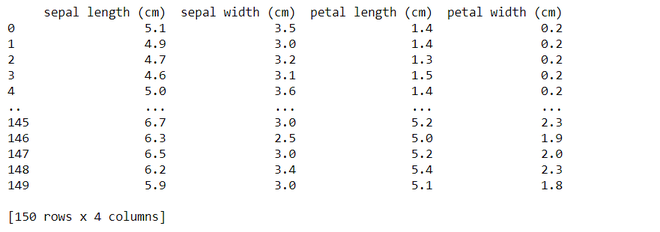### There are4 methods to Print the entire pandas Dataframe:

• Use to_string() Method
• Use pd.option_context() Method
• Use pd.set_options() Method
• Use pd.to_markdown() Method

### Method 1: Using to_string()

While this method is simplest of all, it is not advisable for very huge datasets (in order of millions) because it converts the entire data frame into a string object but works very well for data frames for size in the order of thousands.

Syntax: DataFrame.to_string(buf=None, columns=None, col_space=None, header=True, index=True, na_rep=’NaN’, formatters=None, float_format=None, index_names=True, justify=None, max_rows=None, max_cols=None, show_dimensions=False, decimal=’.’, line_width=None)

Example: In this example, we are using the `load_iris` function from scikit-learn to load the Iris dataset, then creates a pandas DataFrame (`df`) containing the dataset features, and finally, converts the entire DataFrame to a string representation using `to_string()` and displays it.

## Python3

 `import` `numpy as np` `from` `sklearn.datasets ``import` `load_iris` `import` `pandas as pd`   `data ``=` `load_iris()` `df ``=` `pd.DataFrame(data.data,` `                  ``columns ``=` `data.feature_names)`   `# Convert the whole dataframe as a string and display` `display(df.to_string())`

Output: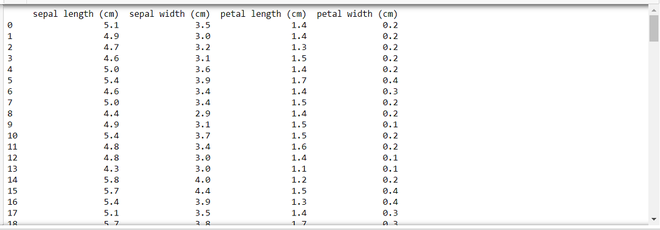### Method 2: Using pd.option_context()

Pandas allow changing settings via the option_context() method and set_option() methods. Both the methods are identical with one difference that later one changes the settings permanently and the former do it only within the context manager scope.

Syntax : pandas.option_context(*args)

Example: In this example, we are using the Iris dataset from scikit-learn, creates a pandas DataFrame (`df`) with specified formatting options, and prints the DataFrame within a temporary context where display settings, such as maximum rows, columns, and precision, are modified for local scope only.

## Python3

 `import` `numpy as np` `from` `sklearn.datasets ``import` `load_iris` `import` `pandas as pd`   `data ``=` `load_iris()` `df ``=` `pd.DataFrame(data.data, ` `                  ``columns ``=` `data.feature_names)`   `# The scope of these changes made to` `# pandas settings are local to with statement.` `with pd.option_context(``'display.max_rows'``, ``None``,` `                       ``'display.max_columns'``, ``None``,` `                       ``'display.precision'``, ``3``,` `                       ``):` `    ``print``(df)`

Output: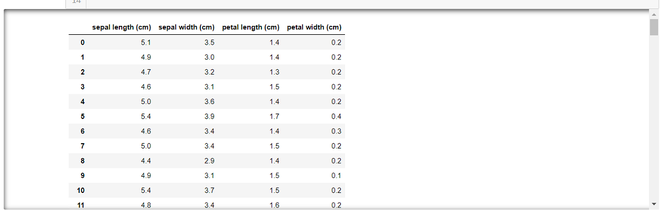### Method 3: Using pd.set_option()

This method is similar to pd.option_context() method and takes the same parameters as discussed for method 2, but unlike pd.option_context() its scope and effect is on the entire script i.e all the data frames settings are changed permanently

To explicitly reset the value use pd.reset_option(‘all’) method has to be used to revert the changes.

Syntax : pandas.set_option(pat, value)

Example: This code modifies global pandas display options to show all rows and columns with unlimited width and precision for the given DataFrame (`df`). It then resets the options to their default values and displays the DataFrame again, illustrating the restoration of default settings.

## Python3

 `import` `numpy as np` `from` `sklearn.datasets ``import` `load_iris` `import` `pandas as pd`   `data ``=` `load_iris()` `df ``=` `pd.DataFrame(data.data,` `                  ``columns ``=` `data.feature_names)`   `# Permanently changes the pandas settings` `pd.set_option(``'display.max_rows'``, ``None``)` `pd.set_option(``'display.max_columns'``, ``None``)` `pd.set_option(``'display.width'``, ``None``)` `pd.set_option(``'display.max_colwidth'``, ``-``1``)`   `# All dataframes hereafter reflect these changes.` `display(df)`   `print``(``'**RESET_OPTIONS**'``)`   `# Resets the options` `pd.reset_option(``'all'``)` `display(df)`

Output: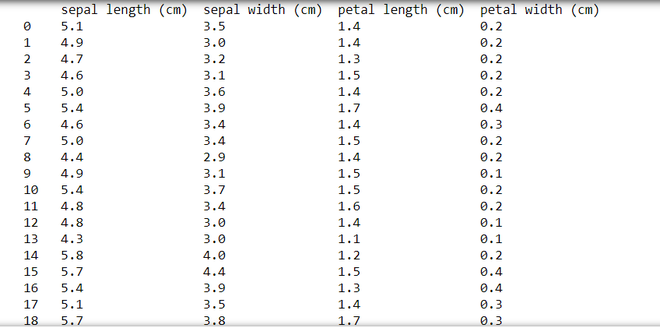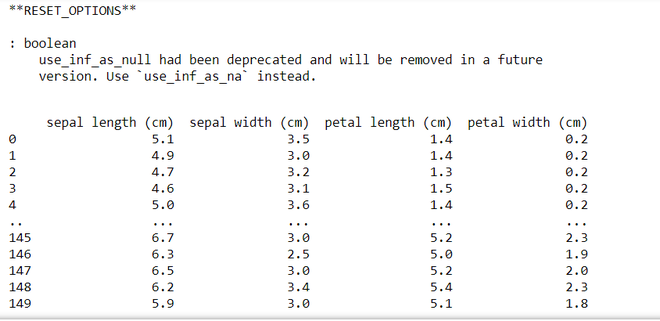### Method 4: Using to_markdown()

This method is similar to the to_string() method as it also converts the data frame to a string object and also adds styling & formatting to it.

Syntax : DataFrame.to_markdown(buf=None, mode=’wt’, index=True,, **kwargs)

Example: This code uses the Iris dataset from scikit-learn to create a pandas DataFrame (`df`), and then it prints a formatted Markdown representation of the DataFrame using the `to_markdown()` method.

## Python3

 `import` `numpy as np` `from` `sklearn.datasets ``import` `load_iris` `import` `pandas as pd`   `data ``=` `load_iris()` `df ``=` `pd.DataFrame(data.data,` `                  ``columns``=``data.feature_names)`   `# Converts the dataframe into str object with formatting` `print``(df.to_markdown())`

Output: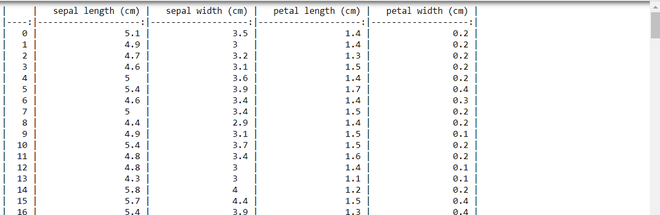Whether you're preparing for your first job interview or aiming to upskill in this ever-evolving tech landscape, GeeksforGeeks Courses are your key to success. We provide top-quality content at affordable prices, all geared towards accelerating your growth in a time-bound manner. Join the millions we've already empowered, and we're here to do the same for you. Don't miss out - check it out now!

Previous
Next